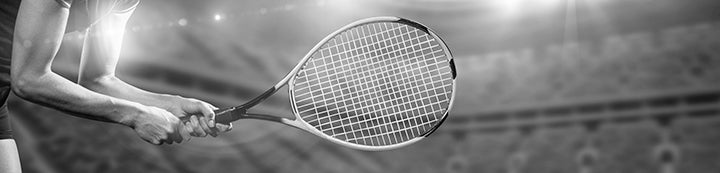21 - 24 of 24 Posts

#### Chilenaitor

·
##### Registered
Joined
·
12,068 Posts
Piter d. Min
Hozumi d. Plipuech
Naydenova d. Lykina

#### .Andrew.

·
##### Registered
Joined
·
18,846 Posts
QFR Results
Piter d. Kalashnikova
Kumhum d. Voracova
Hozumi d. Chang
Rus d. Cako

#### .Andrew.

·
##### Registered
Joined
·
18,846 Posts
Results
Aerosmash: 0+5+7+5= 17
Happydayhk: 0+5+7+5= 17

Meelis: 5+2+4+5= 16
Markmen: 2+5+7+2= 16
goOudinUSA: 2+2+5+7= 16
Szymon: 2+2+7+5= 16

.Andrew.: 2+2+4+7= 15

Silvamarius: 4+4+5+0= 14
Drake1980: 2+5+7+0= 14

Chilenaitor: 5+2+5+0= 12
Windsongs: 0+5+7+0= 12

Wazza: 0+2+7+2= 11
Tennisfan06: 2+2+7+0= 11
Zenith: 0+2+7+2= 11

Randy: 2+2+4+2= 10

Hugues Daniel: 2+5+2+0= 9

F4CU: 0+2+4+2= 8
Mattbrowne2001: 2+2+4+0= 8

Daniela-is-mine: 0+2+2+0= 4

#### .Andrew.

·
##### Registered
Joined
·
18,846 Posts
Congrats @aerosmash and @happydayhk on your 1st and 2nd titles :yeah:

Please check your scores21 - 24 of 24 Posts